# Dividend Sleuthing with R

by Jonathan Regenstein

Welcome to a mid-summer edition of Reproducible Finance with R. Today, we’ll explore the dividend histories of some stocks in the S&P 500. By way of history for all you young tech IPO and crypto investors out there: way back, a long time ago in the dark ages, companies used to take pains to generate free cash flow and then return some of that free cash to investors in the form of dividends. That hasn’t been very popular in the last 15 years, but now that it looks increasing likely that interest rates will never, ever, ever rise to “normal” levels again, dividend yields from those dinosaur companies should be an attractive source of cash for a while.

library(tidyverse)
library(tidyquant)
library(riingo)

riingo_set_token("your tiingo api key here")

We are going to source our data from tiingo by way of the riingo package, the same as we did in this previous post, but first we need the tickers from the S&P 500. Fortunately, the tidyquant package has this covered with the tq_index() function.

sp_500 <-
tq_index("SP500")

sp_500 %>%
head()
# A tibble: 6 x 5
symbol company                     weight sector              shares_held
<chr>  <chr>                        <dbl> <chr>                     <dbl>
1 MSFT   Microsoft Corporation       0.0425 Information Techno…    85542230
2 AAPL   Apple Inc.                  0.0354 Information Techno…    48795028
3 AMZN   Amazon.com Inc.             0.0327 Consumer Discretio…     4616564
4 FB     Facebook Inc. Class A       0.0190 Communication Serv…    26820356
5 BRK.B  Berkshire Hathaway Inc. Cl… 0.0169 Financials             21622312
6 JNJ    Johnson & Johnson           0.0151 Health Care            29639160

We want to pull() out the symbols, and we also want to make sure they are supported by tiingo. We can create a master list of supported tickers with the supported_tickers() function from riingo.

# heads up, there's ~ 79,000 tickers == 4mb of RAM of your machine
test_tickers <-
supported_tickers() %>%
select(ticker) %>%
pull()

Let’s arrange the sp_500 tickers by the weight column and then slice the top 30 for today’s illustrative purposes. We could easily extend this to the entire index by commenting out the slice() code and running this on all 505 tickers (caution: I did this to test the code flow and it works but it’s a big, RAM-intensive job.)

tickers <-
sp_500 %>%
arrange(desc(weight)) %>%
# We'll run this on the top 30, easily extendable to whole 500
slice(1:30) %>%
filter(symbol %in% test_tickers) %>%
pull(symbol)

Let’s import our data from tiingo, using the riingo package. Since we’re only halfway through 2019 and companies haven’t completed their annual dividend payments yet, let’s exclude this year and set end_date = "2018-12-31".

divs_from_riingo <-
tickers %>%
riingo_prices(start_date = "1990-01-01", end_date = "2018-12-31") %>%
arrange(ticker) %>%
mutate(date = ymd(date))

divs_from_riingo %>%
select(date, ticker, close, divCash) %>%
head()
# A tibble: 6 x 4
date       ticker close divCash
<date>     <chr>  <dbl>   <dbl>
1 1990-01-02 AAPL    37.2       0
2 1990-01-03 AAPL    37.5       0
3 1990-01-04 AAPL    37.6       0
4 1990-01-05 AAPL    37.8       0
5 1990-01-08 AAPL    38         0
6 1990-01-09 AAPL    37.6       0

Let’s take a look at the most recent dividend paid by each company, to search for any weird outliers. We don’t want any 0-dollar dividend dates, so we filter(divCash > 0), and we get the most recent payment with slice(n()), which grabs the last row of each group.

divs_from_riingo %>%
group_by(ticker) %>%
filter(divCash > 0) %>%
slice(n()) %>%
ggplot(aes(x = date, y = divCash, color = ticker)) +
geom_point() +
geom_label(aes(label = ticker)) +
scale_y_continuous(labels = scales::dollar)  +
scale_x_date(breaks = scales::pretty_breaks(n = 10)) +
labs(x = "", y = "div/share", title = "2019 Divs: Top 20 SP 500 companies") +
theme(legend.position = "none",
plot.title = element_text(hjust = 0.5))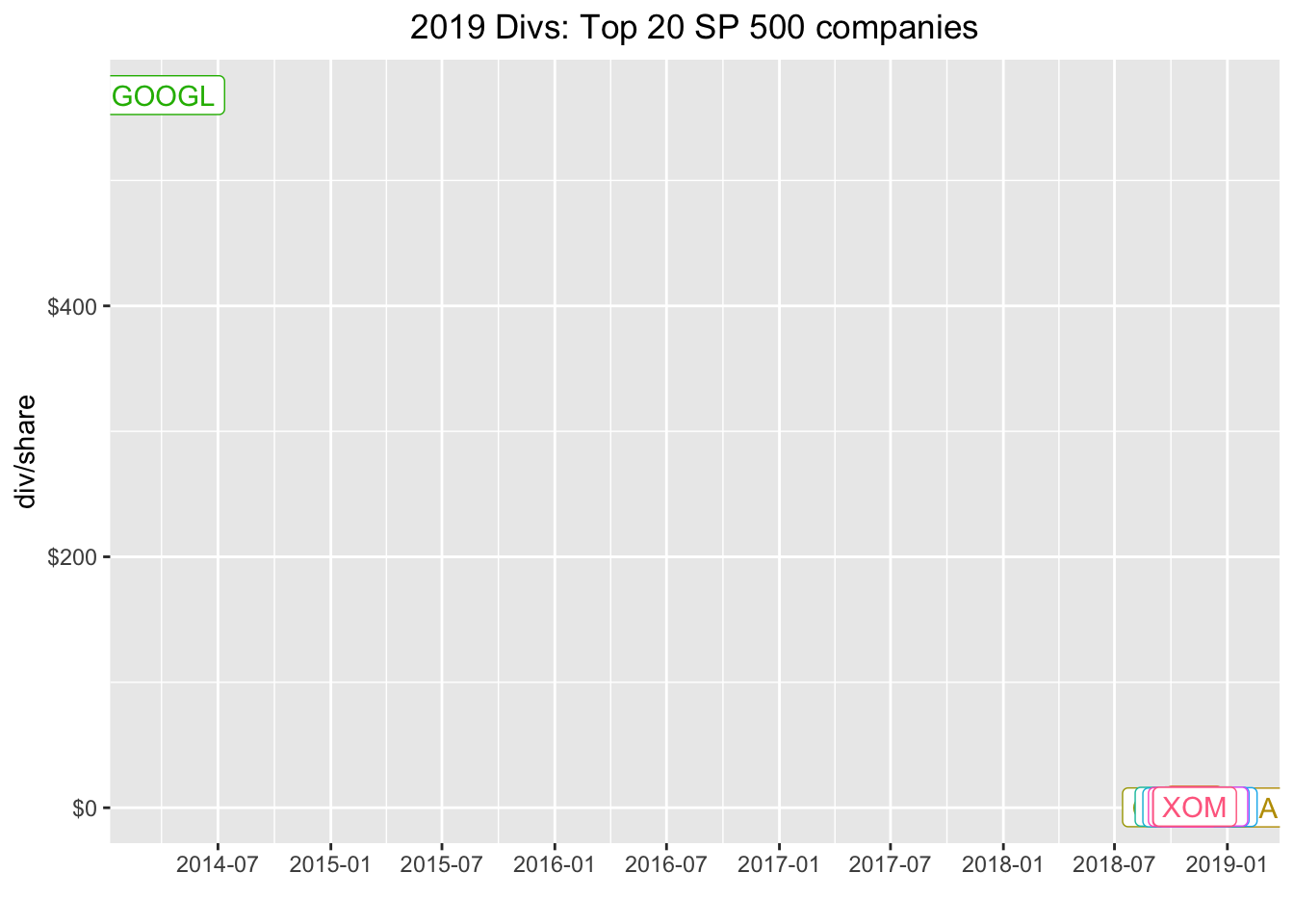A $500 dividend from Google back in 2014? A little, um, internet search engine’ing reveals that Google had a stock split in 2014 and issued that split as a dividend. That’s not quite what we want to capture today - in fact, we’re pretty much going to ignore splits and special dividends. For now, let’s adjust our filter to filter(date > "2017-12-31" & divCash > 0) and grab the last dividend paid in 2018. divs_from_riingo %>% group_by(ticker) %>% filter(date > "2017-12-31" & divCash > 0) %>% slice(n()) %>% ggplot(aes(x = date, y = divCash, color = ticker)) + geom_point() + geom_label(aes(label = ticker)) + scale_y_continuous(labels = scales::dollar) + scale_x_date(breaks = scales::pretty_breaks(n = 10)) + labs(x = "", y = "div/share", title = "2018 Divs: Top 20 SP 500 companies") + theme(legend.position = "none", plot.title = element_text(hjust = 0.5))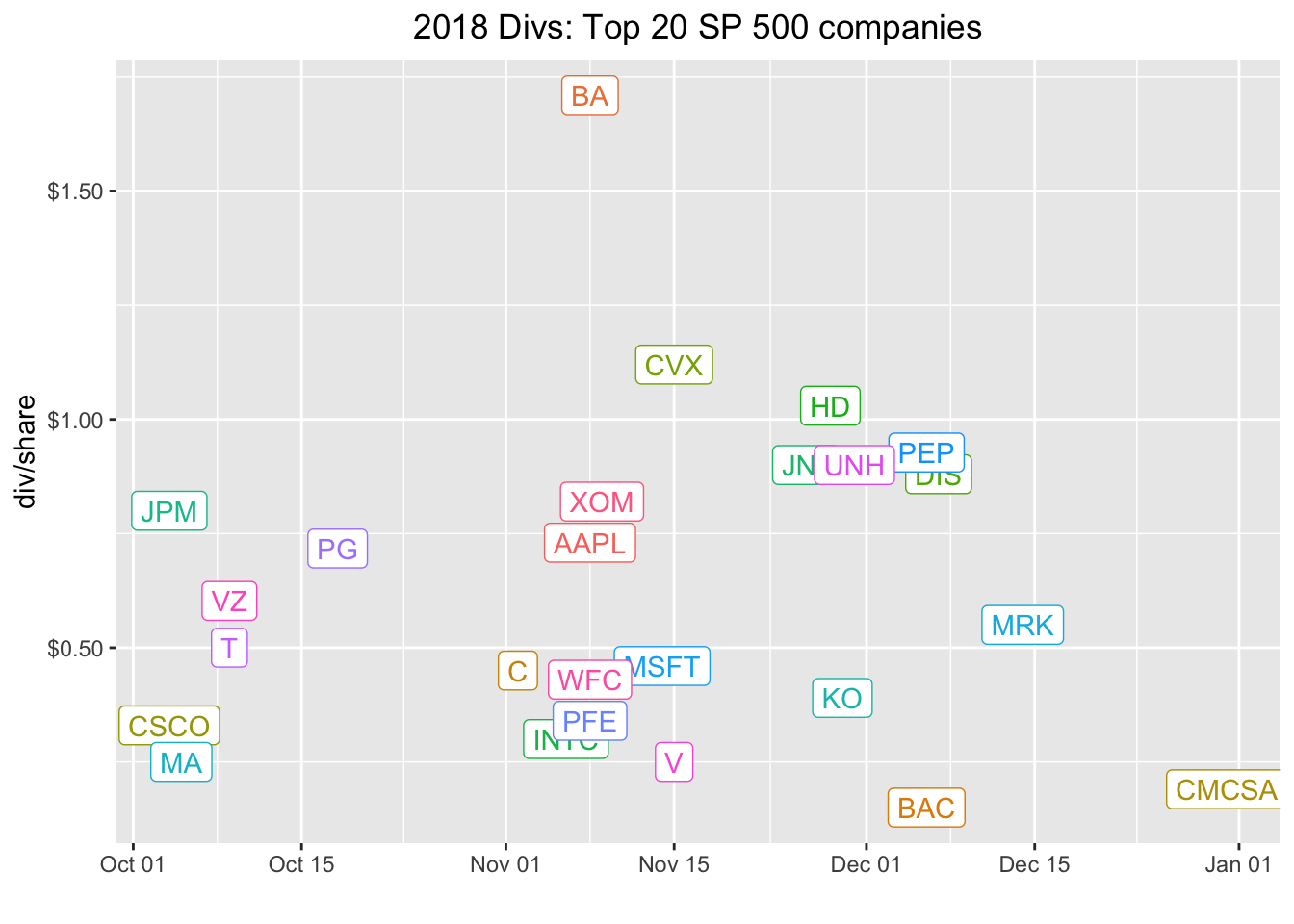Note, this is the absolute cash dividend payout. The dividend yield, the total annual cash dividend divided by the share price, might be more meaningful to us. To get the total annual yield, we want to sum the total dividends in 2018 and divide by the closing price at, say, the first dividend date. To get total dividends in a year, we first create a year column with mutate(year = year(date)), then group_by(year, ticker), and finally make the calculation with mutate(div_total = sum(divCash)). From there, the yield is mutate(div_yield = div_total/close). divs_from_riingo %>% group_by(ticker) %>% filter(date > "2017-12-31" & divCash > 0) %>% mutate(year = year(date)) %>% group_by(year, ticker) %>% mutate(div_total = sum(divCash)) %>% slice(1) %>% mutate(div_yield = div_total/close) %>% ggplot(aes(x = date, y = div_yield, color = ticker)) + geom_point() + geom_text(aes(label = ticker), vjust = 0, nudge_y = 0.002) + scale_y_continuous(labels = scales::percent, breaks = scales::pretty_breaks(n = 10)) + scale_x_date(breaks = scales::pretty_breaks(n = 10)) + labs(x = "", y = "yield", title = "2018 Div Yield: Top 30 SP 500 companies") + theme(legend.position = "none", plot.title = element_text(hjust = 0.5))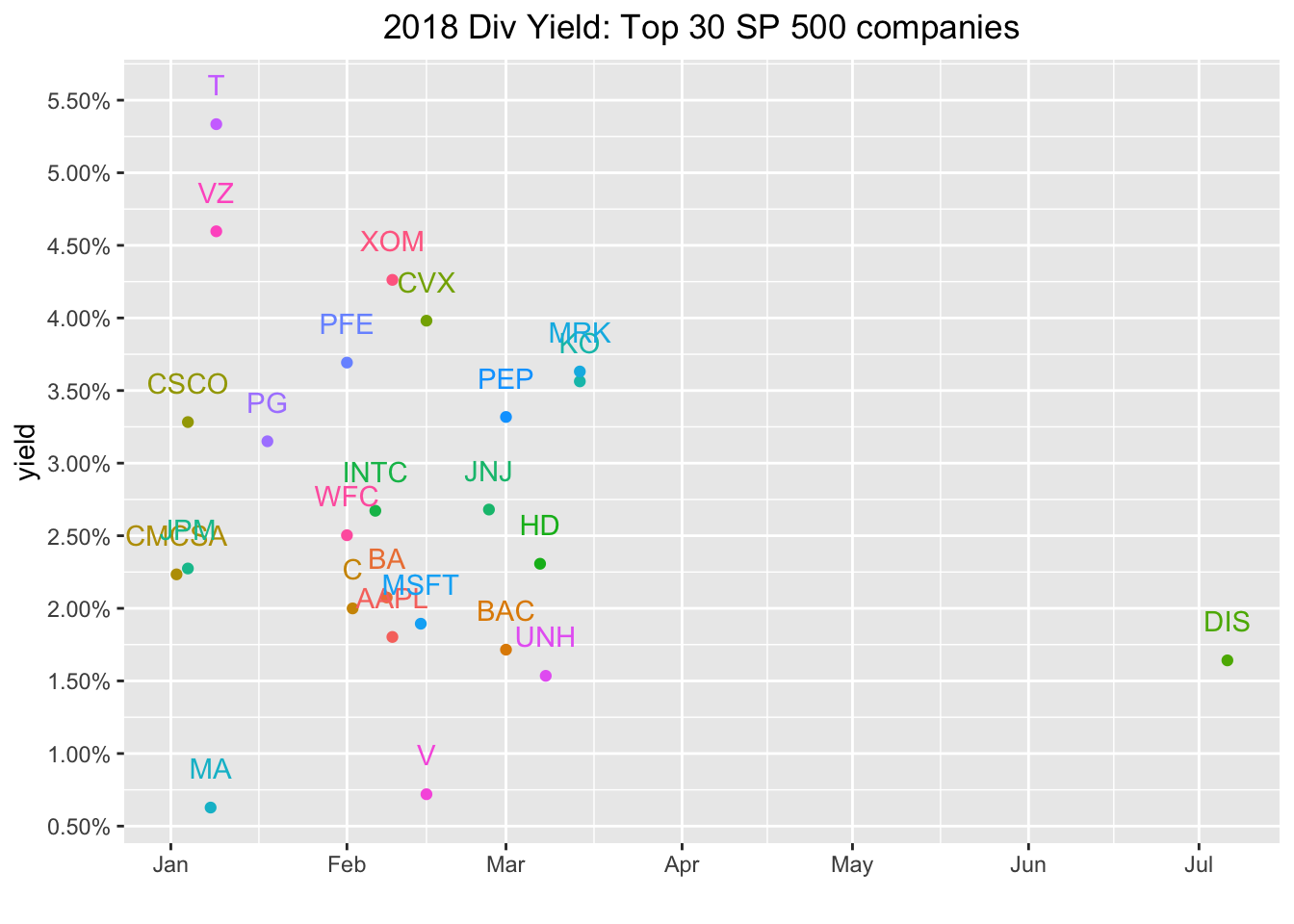Let’s nitpick this visualization and take issue with the fact that some of the labels are overlapping - just the type of small error that drives our end audience crazy. It is also a good opportunity to explore the ggrepel package. We can use the geom_text_repel() function, which will somehow “automatically” position our labels in a non-overlapping way. library(ggrepel) divs_from_riingo %>% group_by(ticker) %>% filter(date > "2017-12-31" & divCash > 0) %>% mutate(year = year(date)) %>% group_by(year, ticker) %>% mutate(div_total = sum(divCash)) %>% slice(1) %>% mutate(div_yield = div_total/close) %>% ggplot(aes(x = date, y = div_yield, color = ticker)) + geom_point() + geom_text_repel(aes(label = ticker), vjust = 0, nudge_y = 0.002) + scale_y_continuous(labels = scales::percent, breaks = scales::pretty_breaks(n = 10)) + scale_x_date(breaks = scales::pretty_breaks(n = 10)) + labs(x = "", y = "yield", title = "2018 Divs: Top 20 SP 500 companies") + theme(legend.position = "none", plot.title = element_text(hjust = 0.5))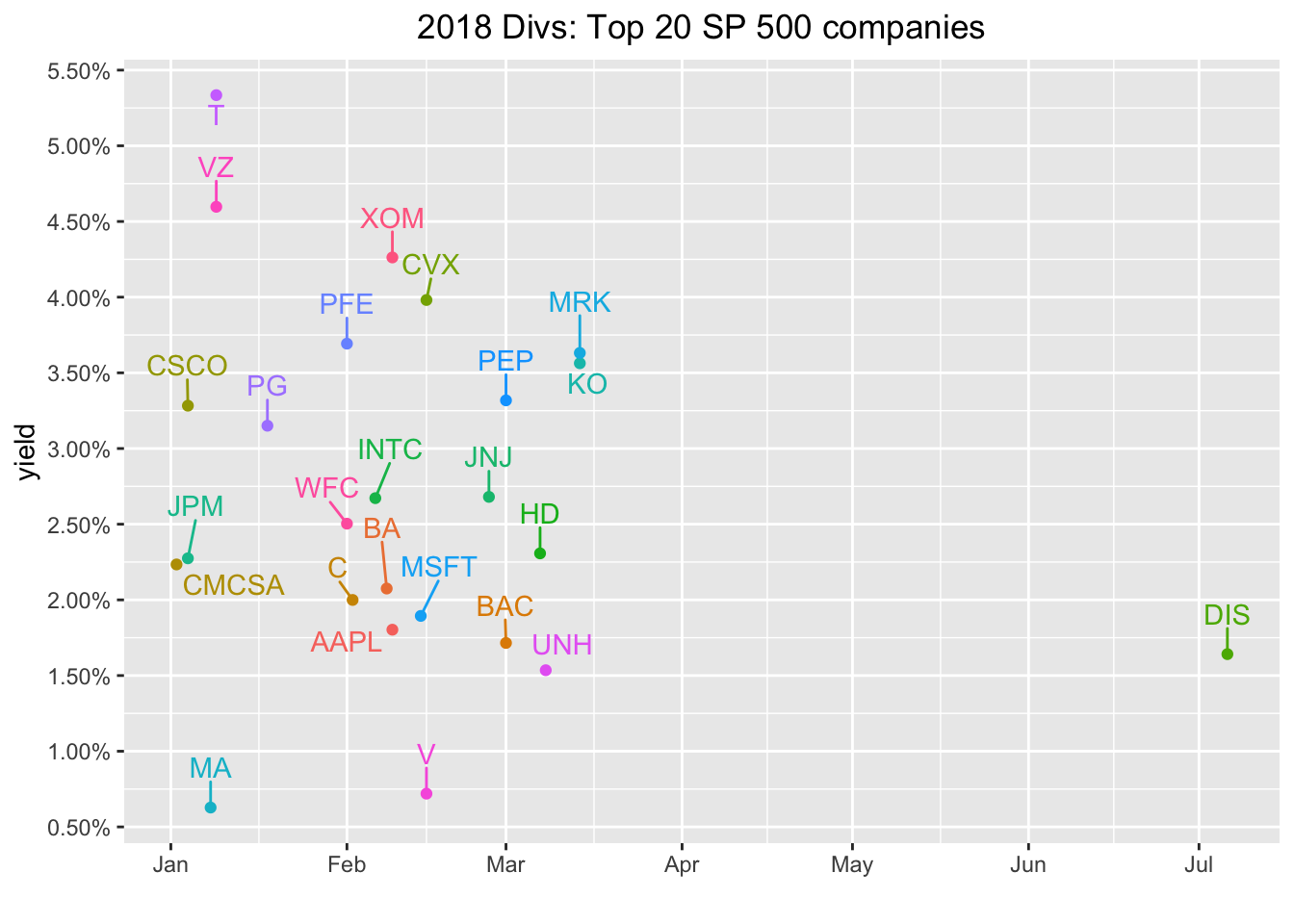We have a decent snapshot of these companies’ dividends in 2018. Let’s dig into their histories a bit. If you’re into this kind of thing, you might have heard of the Dividend Aristocrats or Dividend Kings or some other nomenclature that indicates a quality dividend-paying company. The core of these classifications is the consistency with which companies increase their dividend payouts, because those annual increases indicate a company that has strong free cash flow and believes that shareholder value is tied to the dividend. For examples of companies which don’t fit this description, check out every tech company that has IPO’d in the last decade. (Just kidding. Actually, I’m not kidding but now I guess I’m obligated to do a follow-up post on every tech company that has IPO’d in the last decade so we can dig into their free cash flow - stay tuned!) Back to dividend consistency, we’re interested in how each company has behaved each year, and specifically scrutinizing whether the company increased its dividend from the previous year. This is one of those fascinating areas of exploration that is quite easy to conceptualize and even describe in plain English, but turns out to require (at least for me) quite a bit of thought and white-boarding (I mean Goggling and Stack Overflow snooping) to implement with code. In English, we want to calculate the total dividend payout for each company each year, then count how many years in a row the company has increased that dividend consecutively up to today. Let’s get to it with some code. We’ll start with a very similar code flow as above, except we want the whole history, no filtering to just 2018. Let’s also arrange(ticker) so we we can glance at how each ticker behaved. divs_from_riingo %>% mutate(year = year(date)) %>% #filter(year > "2017") group_by(year, ticker) %>% mutate(div_total = sum(divCash)) %>% slice(1) %>% arrange(ticker) %>% select(div_total) %>% head(10)  # A tibble: 10 x 3 # Groups: year, ticker  year ticker div_total <dbl> <chr> <dbl> 1 1990 AAPL 0.45 2 1991 AAPL 0.48 3 1992 AAPL 0.48 4 1993 AAPL 0.48 5 1994 AAPL 0.48 6 1995 AAPL 0.48 7 1996 AAPL 0 8 1997 AAPL 0 9 1998 AAPL 0 10 1999 AAPL 0  Let’s save that as an object called divs_total. divs_total <- divs_from_riingo %>% mutate(year = year(date)) %>% group_by(year, ticker) %>% mutate(div_total = sum(divCash)) %>% slice(1) %>% arrange(ticker) %>% select(div_total) The data now looks like we’re ready to get to work. But have a quick peek at the AAPL data above. Notice anything weird? AAPL paid a dividend of $5.30 in 2012, $11.80 in 2013, then $7.28 in 2014, then around \$2.00 going forward. There was a stock split and we probably shouldn’t evaluate that as dividend decrease without adjusting for the split. We don’t have that stock split data here today, but if we were doing this in a more robust way, and maybe over the course of two posts, we would mash in stock split data and adjust the dividends accordingly. For now, we’ll just carry on using the div_total as the true dividend payout.

Now we want to find years of consecutive increase, up to present day. Let’s use mutate(div_increase = case_when(divCash > lag(divCash, 1) ~ 1, TRUE ~ 0)) to create a new column called div_increase that is a 1 if the dividend increased from the previous year and a 0 otherwise.

divs_total %>%
group_by(ticker) %>%
mutate(div_increase = case_when(div_total > lag(div_total, 1) ~ 1,
TRUE ~ 0)) %>%
tail(10)
# A tibble: 10 x 4
# Groups:   ticker 
year ticker div_total div_increase
<dbl> <chr>      <dbl>        <dbl>
1  2009 XOM         1.66            1
2  2010 XOM         1.74            1
3  2011 XOM         1.85            1
4  2012 XOM         2.18            1
5  2013 XOM         2.46            1
6  2014 XOM         2.70            1
7  2015 XOM         2.88            1
8  2016 XOM         2.98            1
9  2017 XOM         3.06            1
10  2018 XOM         3.23            1

We can see that XOM has a nice history of increasing its dividend.

For my brain, it’s easier to work through the next steps if we put 2018 as the first observation. Let’s use arrange(desc(year)) to accomplish that.

divs_total %>%
group_by(ticker) %>%
mutate(div_increase = case_when(div_total > lag(div_total, 1) ~ 1,
TRUE ~ 0)) %>%
arrange(desc(year)) %>%
arrange(ticker) %>%
slice(1:10)
# A tibble: 282 x 4
# Groups:   ticker 
year ticker div_total div_increase
<dbl> <chr>      <dbl>        <dbl>
1  2018 AAPL        2.82            1
2  2017 AAPL        2.46            1
3  2016 AAPL        2.23            1
4  2015 AAPL        2.03            0
5  2014 AAPL        7.28            0
6  2013 AAPL       11.8             1
7  2012 AAPL        5.3             1
8  2011 AAPL        0               0
9  2010 AAPL        0               0
10  2009 AAPL        0               0
# … with 272 more rows

Here I sliced off the first 10 rows of each group, so I could glance with my human eyes and see if anything looked weird or plain wrong. Try filtering by AMZN or FB or GOOG, companies we know haven’t paid a dividend. Make sure we see all zeroes. How about, say, MSFT?

divs_total %>%
group_by(ticker) %>%
mutate(div_increase = case_when(div_total > lag(div_total, 1) ~ 1,
TRUE ~ 0)) %>%
arrange(desc(year)) %>%
arrange(ticker) %>%
filter(ticker == "MSFT")
# A tibble: 29 x 4
# Groups:   ticker 
year ticker div_total div_increase
<dbl> <chr>      <dbl>        <dbl>
1  2018 MSFT        1.72            1
2  2017 MSFT        1.59            1
3  2016 MSFT        1.47            1
4  2015 MSFT        1.29            1
5  2014 MSFT        1.15            1
6  2013 MSFT        0.97            1
7  2012 MSFT        0.83            1
8  2011 MSFT        0.68            1
9  2010 MSFT        0.55            1
10  2009 MSFT        0.52            1
# … with 19 more rows

Looks like a solid history going back to 2003. In 2004 MSFT issued a special dividend that then made 2005 look like a down year. Again, we’re not correcting for splits and special events so we’ll just let this be. Back to it.

Now we want to start in 2018 (this year) and count the number of consecutive dividend increases, or 1’s in the div_increase column, going back in time. This is the piece that I found most difficult to implement - how to detect if a certain behavior has occurred every year, and if we see it stop, we want to delete all the rows after is has stopped? I considered trying to condition on both increases and consecutive years, but finally discovered a combination of slice(), seq_len(), and min(which(div_increase == 0)) that worked well.

Let’s take it piece-by-painstaking-piece. If we just slice(which(div_increase == 0)), we get every instance of a dividend increase equal to 0.

divs_total %>%
group_by(ticker) %>%
mutate(div_increase = case_when(div_total > lag(div_total, 1) ~ 1,
TRUE ~ 0)) %>%
arrange(desc(year)) %>%
arrange(ticker) %>%
slice(which(div_increase == 0))
# A tibble: 319 x 4
# Groups:   ticker 
year ticker div_total div_increase
<dbl> <chr>      <dbl>        <dbl>
1  2015 AAPL        2.03            0
2  2014 AAPL        7.28            0
3  2011 AAPL        0               0
4  2010 AAPL        0               0
5  2009 AAPL        0               0
6  2008 AAPL        0               0
7  2007 AAPL        0               0
8  2006 AAPL        0               0
9  2005 AAPL        0               0
10  2004 AAPL        0               0
# … with 309 more rows

If we use slice(min(which(div_increase == 0))), we get the first instance, for each group, of a dividend increase of zero.

divs_total %>%
group_by(ticker) %>%
mutate(div_increase = case_when(div_total > lag(div_total, 1) ~ 1,
TRUE ~ 0)) %>%
arrange(desc(year)) %>%
arrange(ticker) %>%
slice(min(which(div_increase == 0)))
# A tibble: 29 x 4
# Groups:   ticker 
year ticker div_total div_increase
<dbl> <chr>      <dbl>        <dbl>
1  2015 AAPL       2.03             0
2  2018 AMZN       0                0
3  2011 BA         1.68             0
4  2013 BAC        0.04             0
5  2014 C          0.04             0
6  2017 CMCSA      0.473            0
7  2010 CSCO       0                0
8  2005 CVX        1.75             0
9  2009 DIS        0.35             0
10  2018 FB         0                0
# … with 19 more rows

The magic comes from seq_len. If we use slice(seq_len(min(which(div_increase == 0)))), then we slice the rows from row 1 (which recall is the year 2018) through the first time we see a dividend increase of 0. seq_len() creates a sequence of 1 through some number, in this case, we create a sequence of 1 through the row number where we first see a dividend increase of 0 (there’s a good tutorial on seq_len here). And that’s what we want to keep.

divs_total %>%
group_by(ticker) %>%
mutate(div_increase = case_when(div_total > lag(div_total, 1) ~ 1,
TRUE ~ 0)) %>%
arrange(desc(year)) %>%
arrange(ticker) %>%
slice(seq_len(min(which(div_increase == 0))))
# A tibble: 240 x 4
# Groups:   ticker 
year ticker div_total div_increase
<dbl> <chr>      <dbl>        <dbl>
1  2018 AAPL        2.82            1
2  2017 AAPL        2.46            1
3  2016 AAPL        2.23            1
4  2015 AAPL        2.03            0
5  2018 AMZN        0               0
6  2018 BA          6.84            1
7  2017 BA          5.68            1
8  2016 BA          4.36            1
9  2015 BA          3.64            1
10  2014 BA          2.92            1
# … with 230 more rows

Now let’s order our data by those years of increase, so that the company with the longest consecutive years of increase is at the top.

divs_total %>%
group_by(ticker) %>%
mutate(div_increase = case_when(div_total > lag(div_total, 1) ~ 1,
TRUE ~ 0)) %>%
arrange(desc(year)) %>%
arrange(ticker) %>%
slice(seq_len(min(which(div_increase ==0)))) %>%
mutate(div_inc_consec = sum(div_increase)) %>%
slice(1) %>%
arrange(desc(div_inc_consec))
# A tibble: 29 x 5
# Groups:   ticker 
year ticker div_total div_increase div_inc_consec
<dbl> <chr>      <dbl>        <dbl>          <dbl>
1  2018 PEP         3.59            1             20
2  2018 JNJ         3.54            1             16
3  2018 XOM         3.23            1             16
4  2018 CVX         4.48            1             13
5  2018 MSFT        1.72            1             13
6  2018 PG          2.84            1             13
7  2018 T           2               1             12
8  2018 DIS         1.72            1              9
9  2018 HD          4.12            1              9
10  2018 UNH         3.45            1              9
# … with 19 more rows

And the winner is….

divs_total %>%
group_by(ticker) %>%
mutate(div_increase = case_when(div_total > lag(div_total, 1) ~ 1,
TRUE ~ 0)) %>%
arrange(desc(year)) %>%
arrange(ticker) %>%
slice(seq_len(min(which(div_increase ==0)))) %>%
mutate(div_inc_consec = sum(div_increase)) %>%
slice(1) %>%
arrange(desc(div_inc_consec)) %>%
filter(div_inc_consec > 0) %>%
ggplot(aes(x = reorder(ticker, div_inc_consec), y = div_inc_consec, fill = ticker)) +
geom_col(width = .5) +
geom_label_repel(aes(label = ticker), color = "white", nudge_y = .6) +
theme(legend.position = "none",
axis.title.x = element_blank(),
axis.text.x = element_blank(),
axis.ticks.x = element_blank()) +
labs(x = "", y = "years consec div increase") +
scale_y_continuous(breaks = scales::pretty_breaks(n = 10))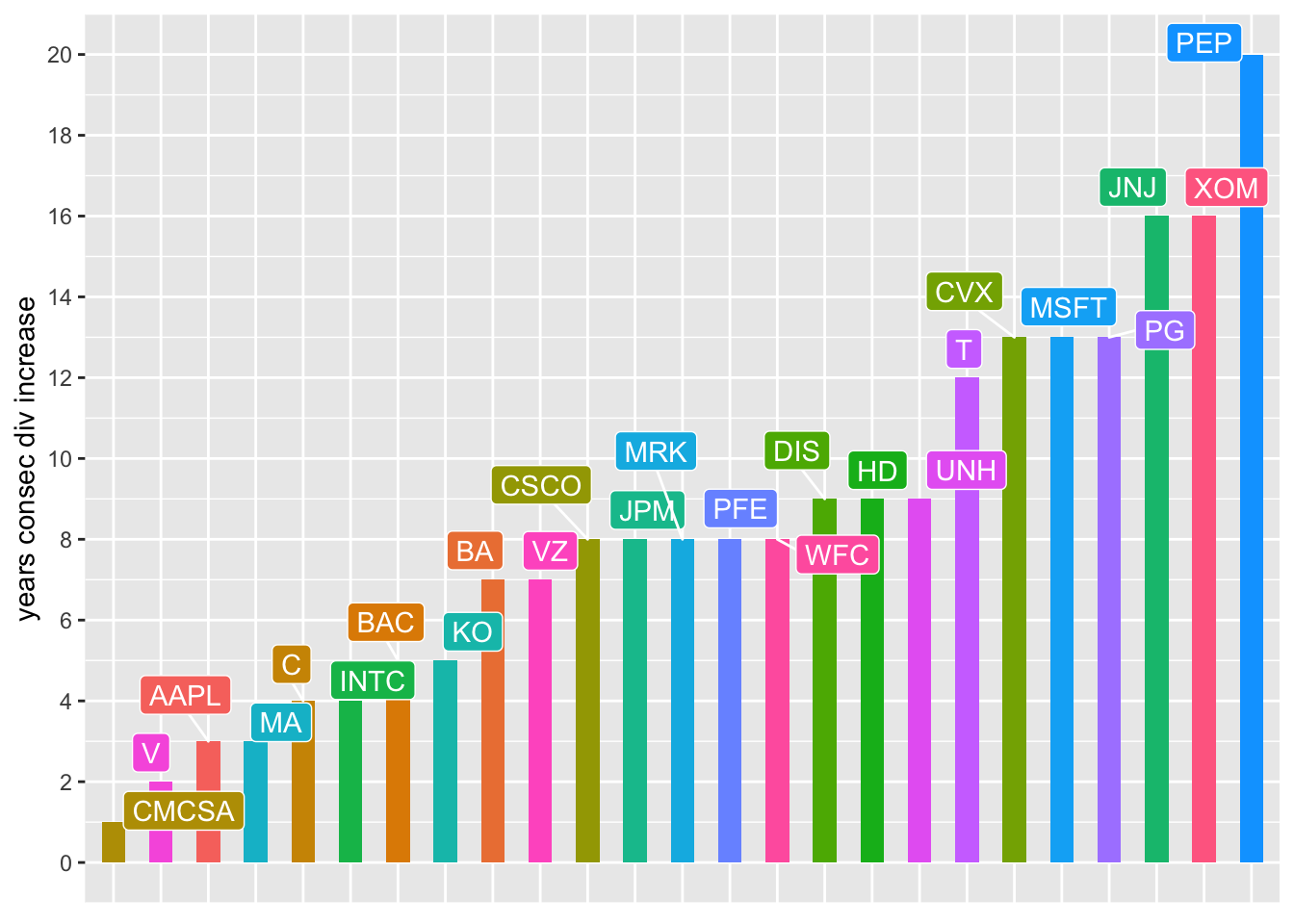PEP!! Followed by JNJ and XOM, though MSFT would have made the leader board without that special dividend, which made their dividend seem to drop in 2005.

Before we close, for the curious, I did run this separately on all 505 members of the S&P 500. It took about five minutes to pull the data from tiingo because it’s 500 tickers, daily data, over 28 years, 2 million rows, 14 columns, and total size of 305.2 MB, but fortes fortuna iuvat. Here’s a plot of all the tickers with at least five consecutive years of dividend increases. This was created with the exact code flow we used above, except I didn’t filter down to the top 30 by market cap.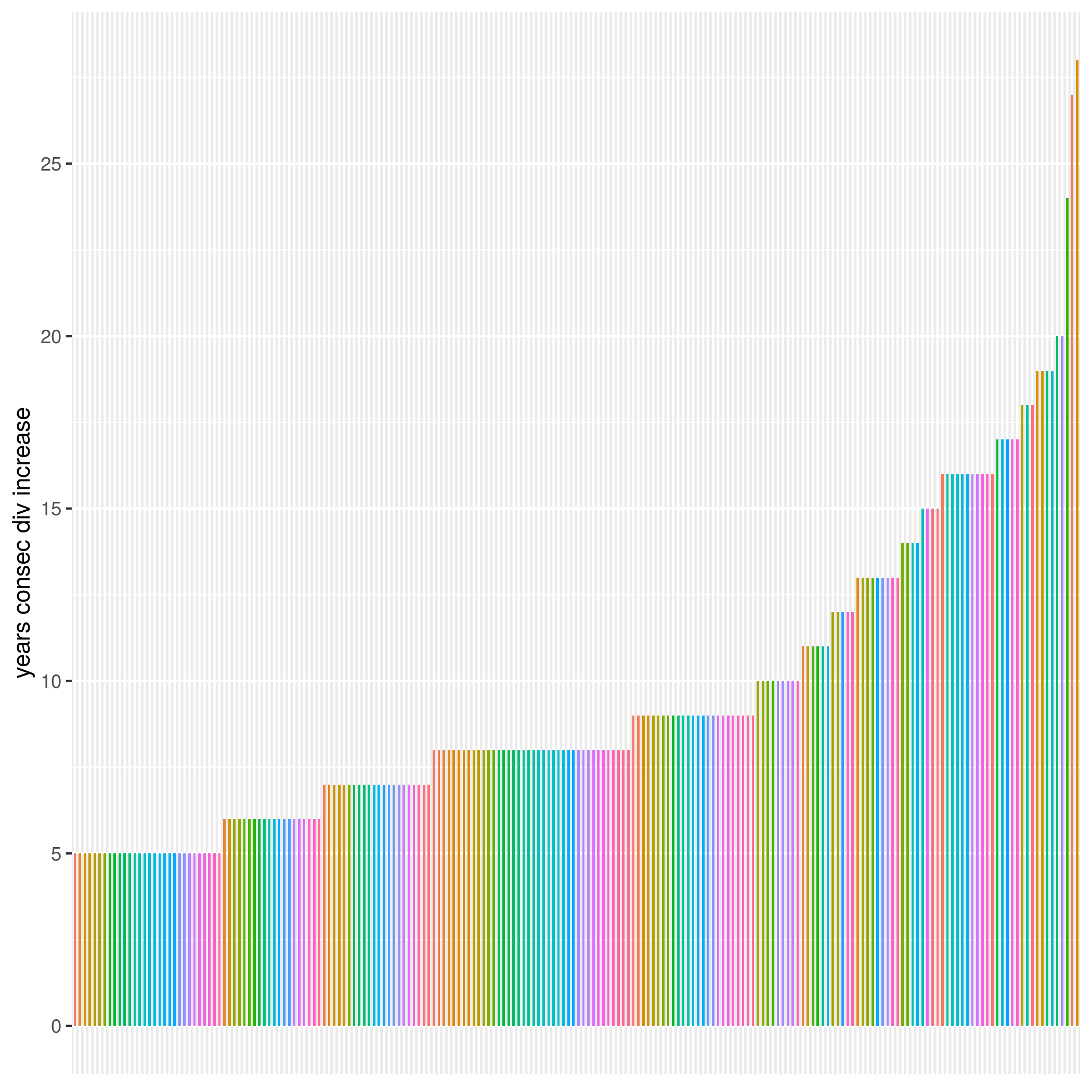Aren’t you just dying to know which ticker is that really high bar at the extreme right? It’s ATO, Atmos Energy Corporation, with 28 years of consecutive dividend increases but, side note, if we had adjusted for stock splits, ATO would not have won this horse race.

A couple of plugs before we close:

If you like this sort of thing check out my book, Reproducible Finance with R.

I’m also going to be posting weekly code snippets on linkedin, connect with me there if you’re keen for some weekly R finance stuff.

Happy coding!

Share Comments · · · ·